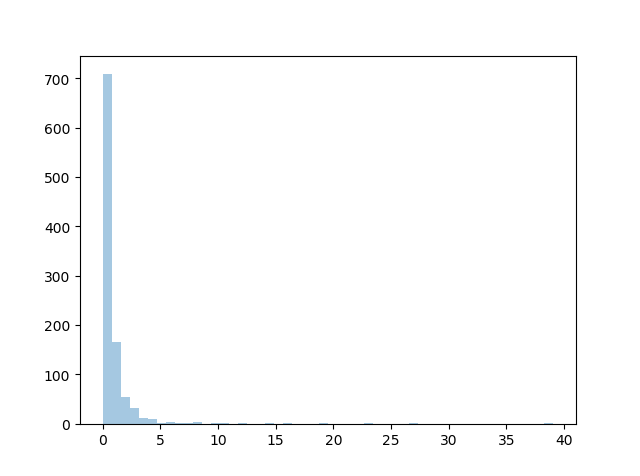# Pareto Distribution

## Pareto Distribution

A distribution following Pareto's law i.e. 80-20 distribution (20% factors cause 80% outcome).

It has two parameter:

`a` - shape parameter.

`size` - The shape of the returned array.

### Example

Draw out a sample for pareto distribution with shape of 2 with size 2x3:

from numpy import random

x = random.pareto(a=2, size=(2, 3))

print(x)
Try it Yourself »

## Visualization of Pareto Distribution

### Example

from numpy import random
import matplotlib.pyplot as plt
import seaborn as sns

sns.distplot(random.pareto(a=2, size=1000), kde=False)

plt.show()

### ResultTry it Yourself »

W3Schools is optimized for learning and training. Examples might be simplified to improve reading and learning. Tutorials, references, and examples are constantly reviewed to avoid errors, but we cannot warrant full correctness of all content. While using W3Schools, you agree to have read and accepted our terms of use, cookie and privacy policy.